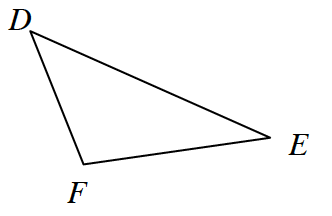### Home > CCG > Chapter 2 > Lesson 2.2.1 > Problem2-67

2-67.Examine the triangle at right.

Review the Math Notes Box in this lesson.

1. If $m∠D=48º$ and $m∠F=117º$, then what is $m∠E$?

Use the Triangle Angle Sum Theorem: $48°+117°+m∠E=180°$

2. Solve for $x$ if $m∠D=4x+2º$, $m∠F=7x-8º$, and $m∠E=4x+6º$. Then find $m∠D$.

Use the same method as part (a).

3. If $m∠D=m∠F=m∠E$, what type of triangle is $ΔFED$?

If all of the angles have equal measure, then all of the sides have equal measure.